##### A java applet to study wave packet dynamics

If you see the applet above, java is installed in your computer; if you don't, you need to download and install java runtime environment (jre) from www.java.com.

###### Wave packet and wave packet dynamics

How does one represent a freely moving particle in quantum mechanics?

Intuitively, one may consider a travelling plane wave represented by a wave-function, which in one dimension is the solution of the Schrodinger time dependent equation with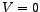: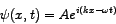where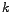and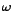can be found out from the relations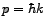and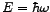. There is a problem though.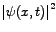then turns out to be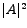, which is constant. This would mean that there is equal probability of the particle to be present anywhere along the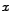-axis, which is unacceptable. Moreover, a wave represented by the above wave function travels in the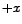direction with the phase velocity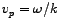. Now, since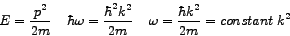(the so called dispersion relation)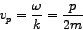which implies that the phase velocity is one-haf of the classical particle velocity.

Thus the above plane wave wave-function does not have any physical significance and as such cannot truely represent a freely moving particle.

Instead, one may take the linear combination of different plane wave wave-functions (with different wave numbers orvalues):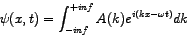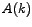is the amplitude of the plane-wave having wave numberor momentum. This superposition, at each instant of time, leads to constructive interference in a small region along-axis and destructive interference outside the region leading to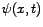being localized inand being spread out inor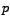. The above wave-function represents what is known as a wave-packet, which in turn truely represents a classically free particle in quantum mechanics. With the change of time, the centre of the wave packet moves with a group velocity: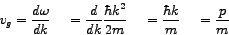which agrees perfectly with the classical velocity of the free particle.

In order to obtainwe find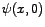: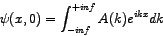is the inverse Fourier transform (IFT) of the function. Therefore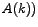= Fourier transform (FT) of.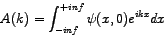We may start with a given, findfrom it and then use it to findwhich would then give us the complete temporal evolution of the wave-packet.

To illustrate this technique we takeas follows: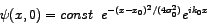The probability density then turns out to be: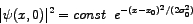which represents a Gaussian distribution.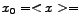mean of the distribution at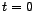and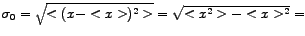standard deviation (square root of the variance) of the distribution at.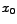also equals the initial position of the center of the wave packet and hence the position of the free particle.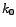corresponds to the initial momentum of the wave-packet. Then we findand then substitute it in the expression for, perform the relevant integration to findand hence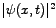. The important observations are as follows:turns out to be a Gaussian distribution too: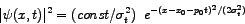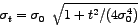Thus the wave-packet localized around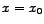atspreads out (broadens or disperses) as it moves with time with group velocity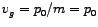. The position of its centre changes with time fromto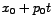. For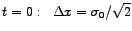For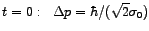Thus for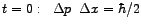i.e. Heisenberg's uncertaity relation is satisfied with our choice of initial wave-packet. Moreover the uncertainty product is minimum. For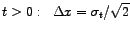For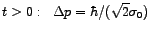i.e. independent of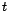. Thus for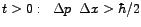. (by making the substitutions)

Hence, Heisenberg's uncertaity relation is again satisfied for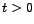. However, the uncertainty product is no more the minimum.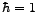and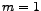throughout if not explicitly mentioned above (atomic units employed).

One may note that plane electromagnetic or light waves and also light pulses (which are wave packets) travelling through vacuum have a linear dispersion relation in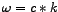(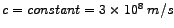). Hence such waves travel without any dispersion. For such plane light waves phase velocity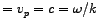and for light pulse wave packets group-velocity is given by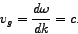However, since dispersion relation of particle wave-packets is non-linear, particle wave packets disperse or broaden with time.

The interactive java applet has been created by me to facilitate the study of the different aspects of the dynamics of a free particle wave packet as well as that of a wave packet tunnelling through a rectangular potential barrier or rectangular potential well. A Gaussian wave packet as described above has been used to launch the simulation.

Instead of following any analytical approach, I have used a numerical approach (The Crank Nicolson implicit method) to solve the relevant time dependent Schrodinger equation.

Input different values in the boxes housed at the top of the applet and press enter to start the simulation. For best results with the applet, keep yourself confined within the following range of values:

0.25 <= x0factor <= 0.75

(x0factor corresponds to the center of the initial wave packet)

- 1.0 < k0factor < 0.0 (for 0.5 <= x0factor <= 0.75)

0.0 < k0factor < 1.0 (for 0.25 <= x0factor <= 0.5)

(k0factor corresponds to the velocity of the initial wave packet)

0.0 < sigma0factor < 0.5

(sigma0factor corresponds to the width of the initial wave packet)

0.0 < barrierwidthfactor < 1.0

(barrierwidthfactor corresponds to the width of the potential barrier)

-1.0 < barrierheightfactor < 1.0

(barrierheightfactor corresponds to the height of the potential barrier; negative values of barrierheightfactor correspond to the case of a rectangular potential well while a zero value corresponds to the motion of a free particle).